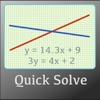# AP Algebra Helper - Lumos Educational App StoreNA1
Price -Free
\$0

#### DESCRIPTION:

This algebra solver allows you to solve for any parameter of linear, parabolic, and dual linear equations. In both numeric and graphical presentations there are convenient sliders to set values of the independent or dependent parameters to help with linear and quadratic formulas. The app has 4 main panels - linear (y = mx+b); quadratic polynomial (y = ax^2 + bx + c); quadratic factored (y = (x+a)(x+b); and dual linear (ay=bx+c, dy=ex+ f). In the front panel there are text entries and sliders for each parameter and a lock button to choose the dependent variable (e.g. the one that is solved).

#### OVERVIEW:

AP Algebra Helper is a free educational mobile app By Radius Development.It helps students practice the following standards .

This page not only allows students and teachers download AP Algebra Helper but also find engaging Sample Questions, Videos, Pins, Worksheets, Books related to the following topics.